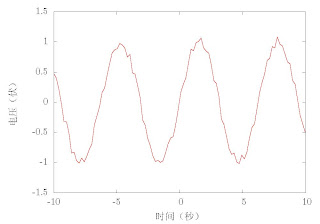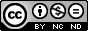## Thursday, December 22, 2011

### Multi-language support of gnuplot --- Using latex (2)

We have shown how to get multi-language support by using latex in the former posts. It works, but a little complicated --- You need to take two steps to get the final graph. In practice, it can be simplified by using an option of the (*)latex terminal. This option is "header". You can put the package use and other commands after this option, and then the generated tex file will contain these information at the header part. So you need not to modify the tex file manually. You get the final graph at one step.

So try the following plot script. Run it and compile the output tex file using latex, and at once you a Chinese supported graph.

reset
set term epslatex color standalone header \
"\\usepackage{CJK}\n \
\\AtBeginDocument{\\begin{CJK*}{GBK}{song}} \
\\AtEndDocument{\\end{CJK*}}"

set output "voltage.tex"

set xlabel "时间（秒）"
set ylabel "电压（伏）"
unset key
plot sin(x)+0.1*(2*rand(0)-1.) w l lw 2
set outputGnuplot plotted graph with Chinese support

## Thursday, December 15, 2011

### gnuplot的多国语言支持----通过latex（1）

reset
set term epslatex color standalone
set output "voltage.tex"
set xlabel "my xlabel"
set ylabel "my ylabel"
unset key
plot sin(x)+0.1*(2*rand(0)-1.) w l lw 2
set output无中文支持的gnuplot绘图结果

\put(4039,154){\makebox(0,0){\strut{}my xlabel}}%


\put(308,2739){\rotatebox{-270}{\makebox(0,0){\strut{}my ylabel}}}


\usepackage{CJK}
\AtBeginDocument{\begin{CJK*}{GBK}{song}}
\AtEndDocument{\end{CJK*}}中文支持的gnuplot绘图结果

voltage.tex  voltage_chinese.tex

## Saturday, December 3, 2011

### Multi-language support of gnuplot --- Using latex

For some non-English speakers, inserting some their own characters into the graph is usual. And for some fresh, this some times becomes a challenging task. This posts will talk about how to solve this problem using latex.

We know that some packages used latex can deal with multi-language typesetting. And we also know that gnuplot have terminals like latex, epslatex. So we can first plot a English graph with these terminals, then modify the output tex files, and at last get a multi-language supported graph. Take Chinese support for example.

We first plot a graph using the following script.

reset
set term epslatex color standalone
set output "voltage.tex"
set xlabel "my xlabel"
set ylabel "my ylabel"
unset key
plot sin(x)+0.1*(2*rand(0)-1.) w l lw 2
set output


Compile the output tex file using latex we get a graph like the following one.Gnuplot plotted graph without Chinese support
And we want to label the x-axes with "时间（秒）"(means time) and y-axes with "电压（伏）"(means voltage). To realize this effect, we open the tex file with any text editor and find lines

\put(4039,154){\makebox(0,0){\strut{}my xlabel}}%


and

\put(308,2739){\rotatebox{-270}{\makebox(0,0){\strut{}my ylabel}}}


Then we repalce "my xlabel" with "时间（秒）", "my ylabel" with "电压（伏）". And add the following codes into the header part of the file to get the chinese support of latex.

\usepackage{CJK}
\AtBeginDocument{\begin{CJK*}{GBK}{song}}
\AtEndDocument{\end{CJK*}}

This time compile the tex file uising latex again. And a chinese supported graph is gotten.Gnuplot plotted graph with Chinese support